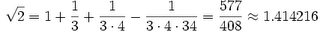SHARE
Baudhāyana, (fl. ca. 800 BC) was an Indian mathematician, who was most likely also a priest. He is noted as the author of the earliest Sulba Sutra — appendices to the Vedas giving rules for the construction of altars — called the Baudhāyana Sulba Sutra, which contained several important mathematical results. He is older to other famous mathematician Apastambha. He belongs to Yajurveda school.

Pythagorean theorem

The most notable of the rules (the Sulbasutras do not contain any proofs of the rules which they describe) in the Baudhāyana Sulba Sutra says:
“A rope stretched along the length of the diagonal produces an area which the vertical and horizontal sides make together.”
This appears to be referring to a rectangle, although some interpretations consider this to refer to a square. In either case, it states that the square of the hypotenuse equals the sum of the squares of the sides. If restricted to right-angled isosceles triangles, however, it would constitute a less general claim, but the text seems to be quite open to unequal sides.

Clearly this is the earliest statement of the what is known today as the Pythagorean theorem.
Baudhayana also provides a non-axiomatic demonstration using a rope measure of the reduced form of the Pythagorean theorem for an isosceles right triangle:
The cord which is stretched across a square produces an area double the size of the original square.
This statement suggests a construction from which the proof of the quoted statement is obvious.

Square root of 2

Baudhayana (elaborated in Apastamba Sulbasutra i.6) gave formula for square root of two:which is correct to five decimals.

Other theorems include: diagonals of rectangle bisect each other, diagonals of rhombus bisect at right angles, area of a square formed by joining the middle points of a square is half of original, the midpoints of a rectangle joined forms a rhombus whose area is half the rectangle, etc.

Note the emphasis on rectangles and squares; this arises from the need to specify yajNa bhUmikAs — i.e. the altar on which a rituals were conducted, including fire offerings (yajNa).

Circling the Square

Another problem tackled by Baudhayana is that of finding a circle whose area is the same as that of a square (the reverse of squaring the circle). His sutra i.58 gives this construction:

Draw half its diagonal about the centre towards the East-West line; then describe a circle together with a third part of that which lies outside the square.

Hope you liked this first in the series post on Great Indian Mathematicians. Let us know what you think.

The Vedic Maths India Team